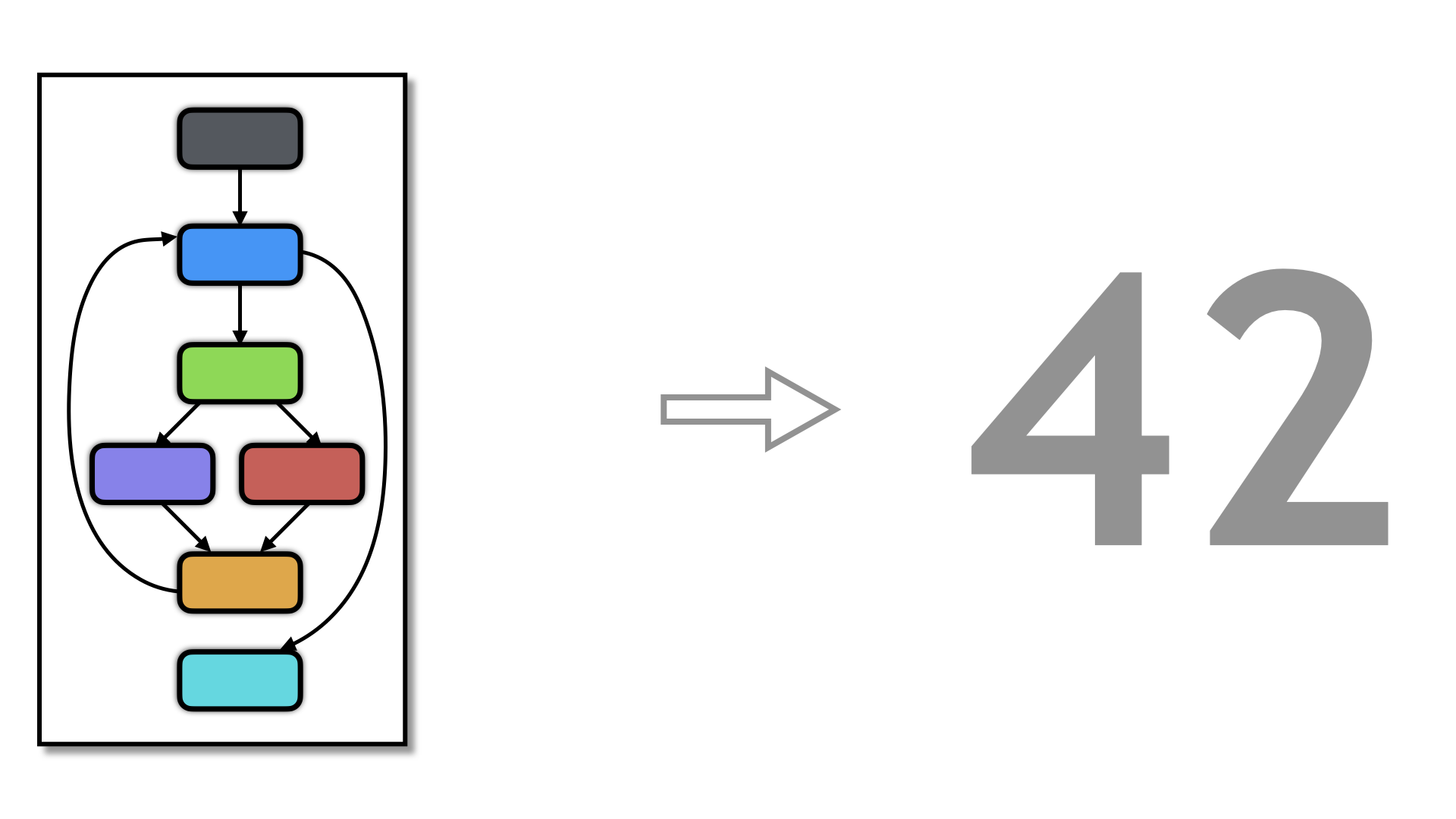# Software Metricsfree web templates

Compute common software complexity metrics. Right now, the Halstead metric (see here and here) and McCabe's cyclomatic complexity metric are available.

OptionArgumentsDescription
--Transform SoftwareMetrics Compute software complexity metrics for a function.
• raw = Raw counts of tokens, identifiers, etc.
• mccabe = McCabe's Cyclomatric Complexity metric.
--SoftwareMetricFileName string On which file to print the metrics. If not set, print to standard output. From version 3.3. Default=Stdout.
--SoftwareMetricJsonFileName string On which file to print the metrics in Json format. From version 3.3. Default=Stdout.

### Example

free web templates

Here is an example where we print out the McCabe metric for three functions: the original fib, fib after flattning, and fib after virtualization. Here is the tigress command:

``````tigress \
--Transform=SoftwareMetrics \
--Functions=fib \
--SoftwareMetricsFileName=original.txt \
--SoftwareMetricsKind=mccabe \
--Transform=Copy \
--Functions=fib \
--CopyName=fib2 \
--Transform=Flatten \
--Functions=fib \
--Transform=SoftwareMetrics \
--Functions=fib \
--SoftwareMetricsFileName=flatten.txt \
--SoftwareMetricsKind=mccabe \
--Transform=Virtualize \
--Functions=fib2 \
--Transform=SoftwareMetrics \
--Functions=fib2 \
--SoftwareMetricsFileName=virtualize.txt \
--SoftwareMetricsKind=mccabe \
test1.c --out=obf.c
``````
free web templates

And here are the results (the variables are the same as seen here and here):

``````> cat original.txt
McCabe.fib.E = 10
McCabe.fib.N = 10
McCabe.fib.P = 1
McCabe.fib.value = 2
> cat flatten.txt
McCabe.fib.E = 28
McCabe.fib.N = 22
McCabe.fib.P = 1
McCabe.fib.value = 8
> cat virtualize.txt
McCabe.fib2.E = 85
McCabe.fib2.N = 68
McCabe.fib2.P = 1
McCabe.fib2.value = 19
``````
free web templates

These metrics can be used to see how much change each transformation incurs.

### Example in Json (From version 3.3)

free web templates

You can also generate the output into a Json file which is useful if you want to process them with some other program:

``````tigress \
--Transform=SoftwareMetrics \
--Functions=wikipedia,sort,main \
--SoftwareMetricsJsonFileName=metrics.json \
test1.c --out=obf.c
``````
``````> cat metrics.json
...
{
"metric": "McCabe",
"name": "sort",
"E": 24,
"N": 21,
"P": 1,
"value": 5
}
...
``````

### Issues

free web templates

Don't expect the values computed by this transform to be identical to that produced by other tools. It only makes sense to compare the metrics computed by Tigress with other metrics computed by Tigress:

• The control flow graphs we build will be different than those produced by other tools - this affects the McCabe metric.
• We're computing token counts over CIL's abstract syntax tree, not over the raw C source. This affects the Halstead metric since CIL performs various simplifications of the code, such as folding while/do/for into one loop.